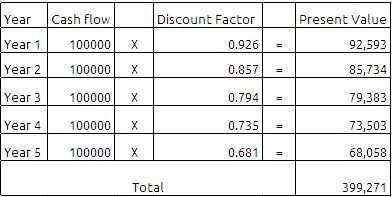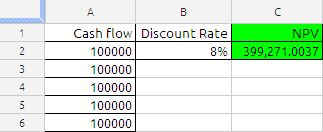## Net Present Value or NPV

The NPV function returns the net present value of an investment based on a series of potentially irregular cash flows that occur at regular time intervals.

Oxford Dictionary of Accounting defines Net Present Value(NPV) as

“A method of capital budgeting in which the value of an investment is calculated as the total present value of all cash inflows and cash outflows minus the cost of initial investment. If the net present value is positive, the return will be greater than that required by the capital markets and investment should be considered. A negative net present value indicates that the investment should be rejected.”

Example:

A Company is considering the purchase of a new machine, which is expected to save 100,000 in cash operating costs each year. Its estimated useful life(how long it will last) is five years, at the end of which it will have no net residual value.

The cash flows for the project are as follows:

Year 0 : Cost of the machine 390,000

Year 1: Savings of 100,000

Year 2: Savings of 100,000

Year 3: Savings of 100,000

Year 4: Savings of 100,000

Year 5: Savings of 100,000

A simple analysis shows that the savings over five years are 500,000 (5 X 100,000) and the cost is 390,000, which gives a surplus of 110,000. However, such an analysis ignores the time value of money; 1 received in future is not equal to 1 received today. To calculate the present value of future cash flows it is necessary to calculate the discount factor.

Assuming that the cost of capital for the project is 8%, the discount factor is calculated as follows:

 Year 1 1/(1.08)^1 Year 2 1/(1.08)^2 Year 3 1/(1.08)^3 Year 4 1/(1.08)^4 Year 5 1/(1.08)^5

The net present value can then be calculated:If we deduct the initial investment of the machine 390,000 then we will get 9,271 which is positive. so we can accept the investment in the project since it is giving the positive cash flow.

All the above is good to understand the background of the Net Present Value (NPV) as far as finance is concerned.

Now we will see how to use this function in google docs spreadsheets.

The Syntax of the function is as follows:

NPV(Rate, value_1, value_2, … value_30)

Rate: The discount rate per period. periodic-discount-rate is a number value and is either entered as a decimal (for example, 0.08) or with a percent sign (for example, 8%). periodic-discount-rate must be greater than or equal to 0.

Value_1, Value_2, …….Value_3 :Values is a number value. A positive value represents income (cash inflow). A negative value represents an expenditure (cash outflow). Value must be equally spaced in time.

Value_1………..: Optionally include one or more additional cash flows.

Usage Notes

periodic-discount-rate is specified using the same time frame as the time frame used for the cash flows. For example, if the cash flows are monthly and the desired annual discount rate is 8%, periodic-discount-rate must be specified as 0.00667 or 0.667% (0.08 divided by 12).

If cash flows are irregular, use the IRR function.

We can achieve the same results using the NPV function as shown in the below image:The formula in cell C2 is

=NPV(B2,A2:A6)